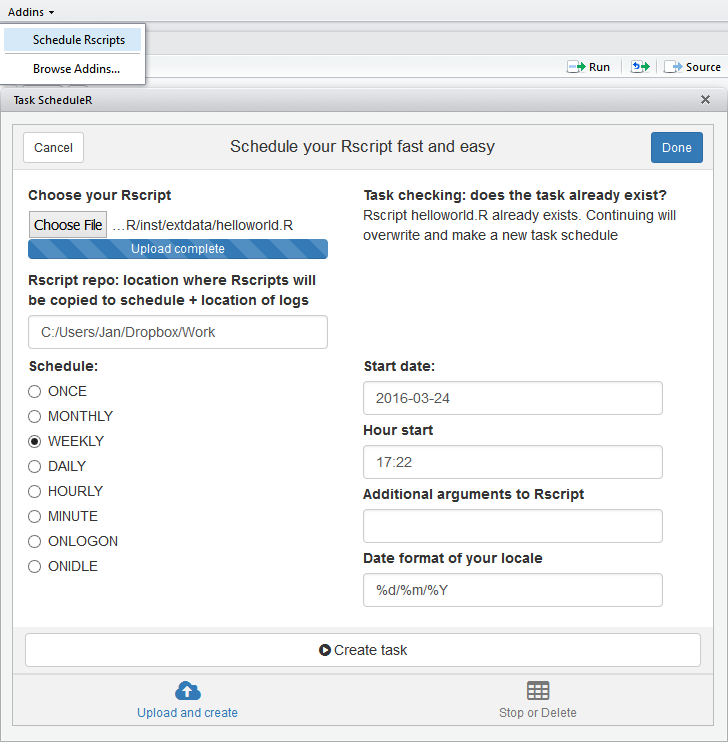#### 2021-04-16Schedule R scripts/processes with the Windows task scheduler. This allows R users working on Windows to automate R processes on specific timepoints from R itself.

## Basic usage

The package allows to

• Get the list of scheduled tasks

• A task is basically a script with R code which is run through Rscript

• You can schedule tasks ‘ONCE’, ‘MONTHLY’, ‘WEEKLY’, ‘DAILY’, ‘HOURLY’, ‘MINUTE’, ‘ONLOGON’, ‘ONIDLE’

• The task log contains the stdout & stderr of the Rscript which was run on that timepoint. This log can be found at the same folder as the R script

Example usage:

library(taskscheduleR)
myscript <- system.file("extdata", "helloworld.R", package = "taskscheduleR")

## run script once within 62 seconds
schedule = "ONCE", starttime = format(Sys.time() + 62, "%H:%M"))

## Run every day at the same time on 09:10, starting from tomorrow on
## Mark: change the format of startdate to your locale if needed (e.g. US: %m/%d/%Y)
schedule = "DAILY", starttime = "09:10", startdate = format(Sys.Date()+1, "%d/%m/%Y"))

## Run every week on Saturday and Sunday at 09:10
schedule = "WEEKLY", starttime = "09:10", days = c('SUN', 'SAT'))

## Run every 5 minutes, starting from 10:40
schedule = "MINUTE", starttime = "10:40", modifier = 5)

## Run every minute, giving some command line arguments
schedule = "MINUTE", rscript_args = "productxyz 20160101")
schedule = "MINUTE", rscript_args = c("productabc", "20150101"))

## get a data.frame of all tasks

taskscheduler_delete(taskname = "myfancyscript_withargs_b")

When the task has run, you can look at the log which contains everything from stdout and stderr. The log file is located at the directory where the R script is located.

## log file is at the place where the helloworld.R script was located
system.file("extdata", "helloworld.log", package = "taskscheduleR")